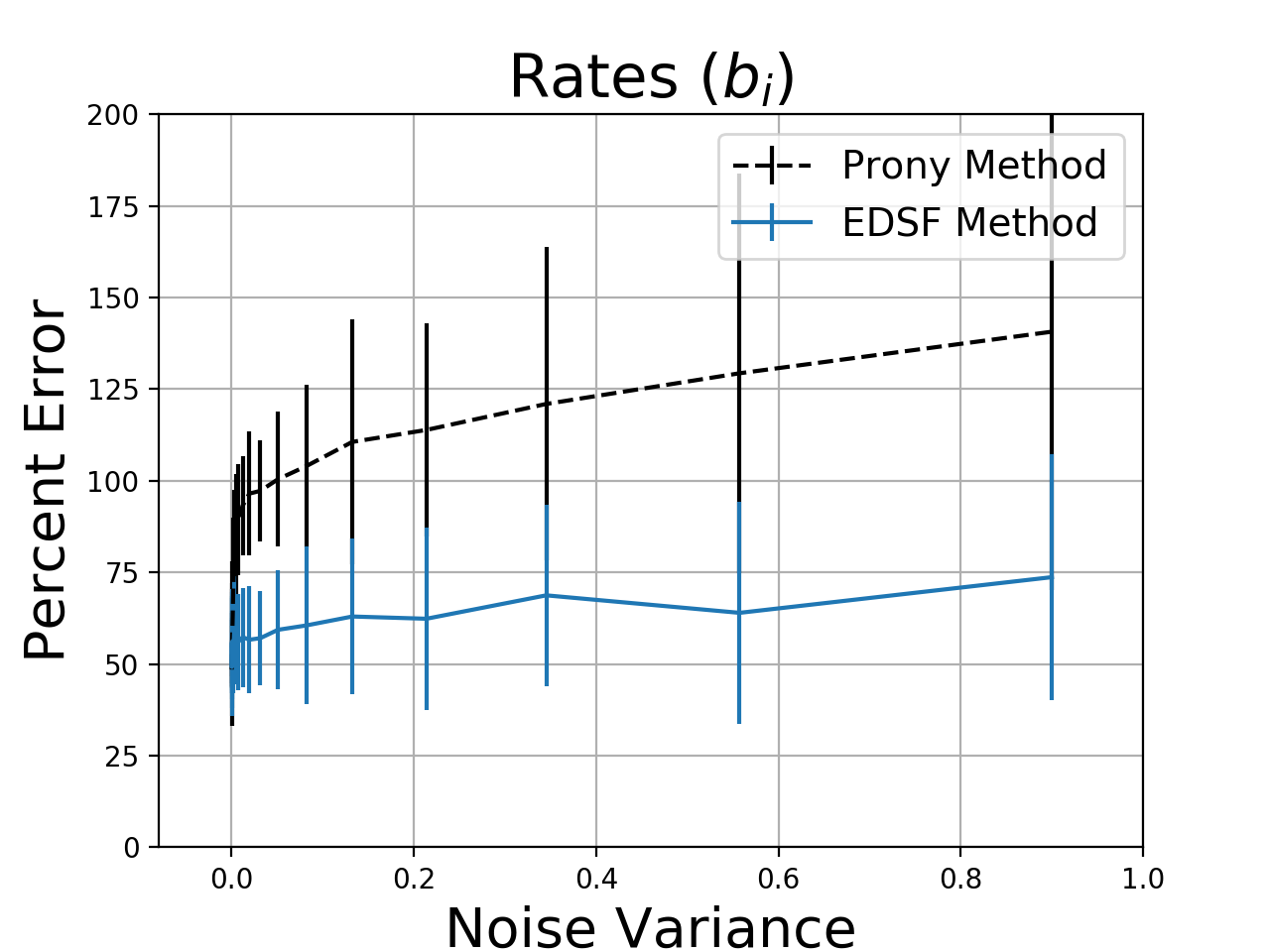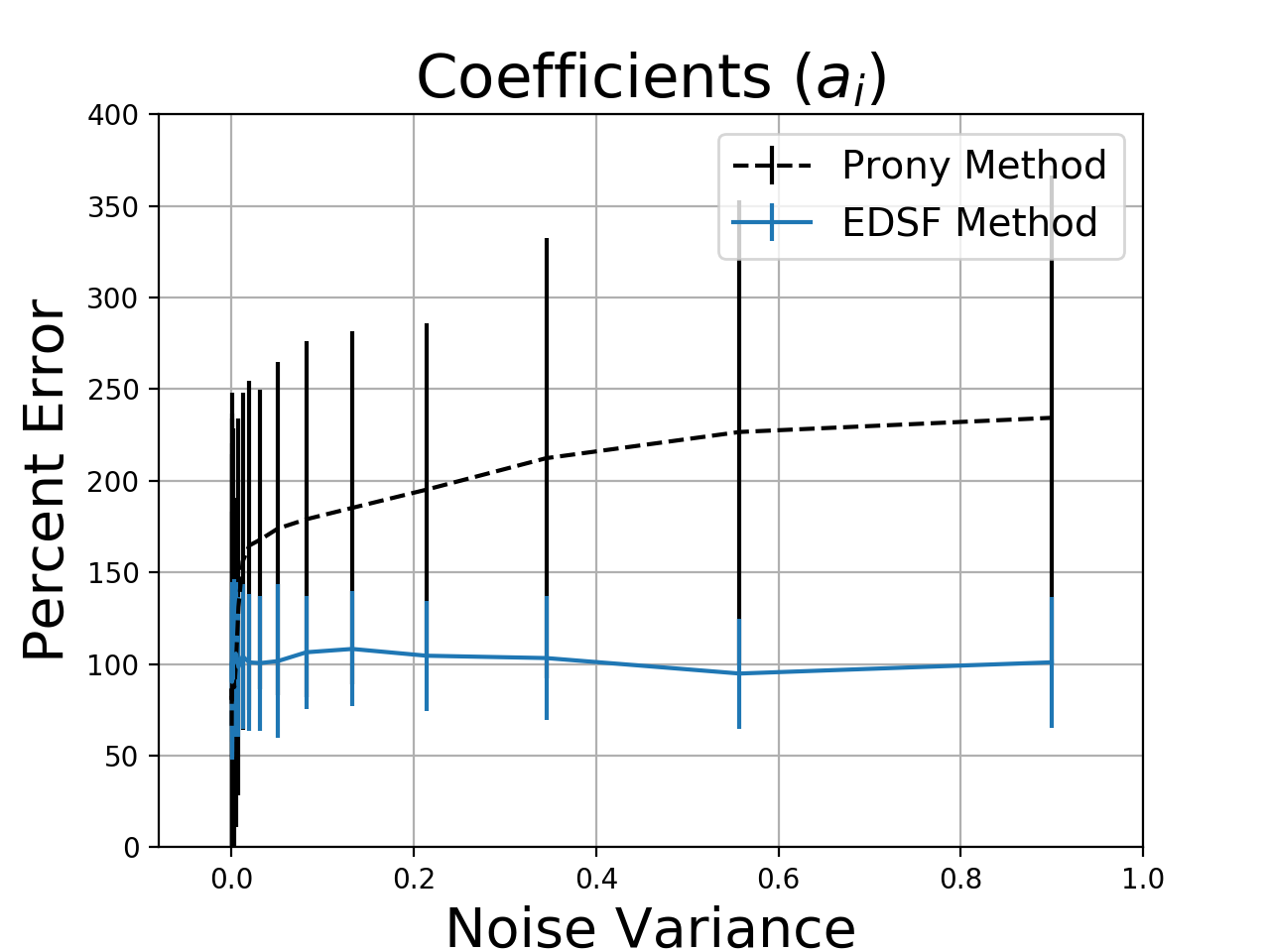# Fitting Exponential Decay Sums with Positive Coefficients

Posted by Ben Coleman on March 08, 2020 · 19 mins read

In this post, we’ll implement a method to fit a sum of exponential decay functions in Python. The code is at the end of the post. Physical scientists encounter the following problem all of the time. We observe a set of values $$y[n]$$ that are generated by multiple exponential decay functions added together and we want to find the individual components of the sum. A climate scientist may do this analyze how different clouds and gasses absorb energy. A chemist may want to identify isotopes in a radioactive sample.

$y[n] = \sum_{i = 1}^{M} a_i e^{b_i n}$

Unfortunately, fitting exponential sums is hard. It’s so hard that Acton, Princeton computer scientist, gives it an entire section of his well-known essay, “What Not to Compute.” It is best to avoid this problem if possible. For instance, if we know the rates $$b_i$$ we can use linear regression instead. If all the $$a_i$$ coefficients are the same, we can take the log to get a softmax and use convex optimization. Even if we don’t know $$a_i$$ or $$b_i$$, it’s a good idea to have as many restrictions as possible to improve our chance of finding a good model. In this post, we’ll deal with the case where $$b_i \leq 0$$ (exponential decay) and $$a_i \geq 0$$ (positive coefficients).

### Prony Method

The Prony method, originally developed in 1795, solves this problem by finding the rates as the roots of a polynomial. Once we have those, we can simply solve for the coefficients via linear regression.

Prony’s method is fast and easy to implement but can misbehave in practice. If we have a sum of decaying exponentials, we do not expect the fitting method to find exponential growth functions or functions that oscillate. Since the rates are found using the roots of a polynomial, $$b_i$$ can be positive, negative or complex-valued. Therefore, Prony’s method can produce sums of sinusoids, exponential growth functions, exponential decay functions, and even complex-valued functions! This is undesirable.

While this algorithm will usually find the right coefficients with noiseless measurements, the situation breaks down rapidly when $$y[n]$$ is contaminated with noise. Noise completely changes the behavior of Prony’s method. Even a relative error of 1% in $$y[n]$$ can be enough to cause serious problems.

### Exponential Decay Sum Fit (EDSF)

It turns out that it is hard to find an algorithm that only fits exponential decay functions with positive coefficients. While there is a lot of theoretical work in this area, it is hard to find a concrete algorithm that can do this. I eventually found a method from a 1977 applied physics paper , which is a practical appliation of the theoretical results found in .

The paper is from 1977, so the computational methods are a little dated. Back then, most computers had less than 16 kB of RAM! The authors put a lot of effort into small optimizations that probably made sense at the time but are unnecessarily complicated today. When I implemented the algorithm, I simplified and eliminated lots of steps.

The algorithm starts by selecting a set of rates $$\theta = e^{b_i}$$ and using linear regression to find the corresponding coefficients $$a_i$$. Then, we carefully remove the terms with negative coefficients from the model. We repeatedly add and remove terms until the algorithm converges. At convergence, we might have more than M terms, so we merge some of the coefficients to output an M-order model.

### Experiment

To compare performance, I generated a 4-term exponential sum with all $$a_i = 1$$ and I used 8 samples of $$y[n]$$. I added various levels of noise to the samples and fitted $$a_i$$ and $$b_i$$ with EDSF and Prony’s method. Since Prony often returns complex coefficients, I used the magnitude of the error between the ground-truth parameters and the fit results to measure the error. I used the Python implementation of Prony’s method from here and the EDSF code from this post.Without noise, Prony’s method is almost exactly correct while EDSF is slightly off. However, as the noise level increases, EDSF more reliably selects parameters that are closer to the true system behavior. Estimation errors of 50% or more are not ideal, but the problem is so sensitive to noise that it is hard to do better. Increasing the number of samples does not help. For both methods, the residual errors were small, but the Prony method produced complex-valued functions and exponential growth about 60% of the time. Since the residual error is small, these techniques might be aceptable for interpolation or modeling even if we cannot reliably recover the true coefficient values.

### Python Code

You can use this function in two ways. If you provide M, the model will keep merging terms until it has only M exponentials left. This is what we used for the experiments. While this method might not produce the best fit, it will result in a low-order model. If you do not provide M, the model will automatically select the correct order. This results in a lower residual error, but may include a few additional terms. We suggest automatic selection because it usually gets the order correct and has a much lower residual error.

 Wiscombe, W. J., and J. W. Evans. “Exponential-sum fitting of radiative transmission functions.” Journal of Computational Physics 24.4 (1977): 416-444.

 Cantor, David G., and John W. Evans. “On approximation by positive sums of powers.” SIAM Journal on Applied Mathematics 18.2 (1970): 380-388.

[Photo Credit] Adrien Olichon on Unsplash